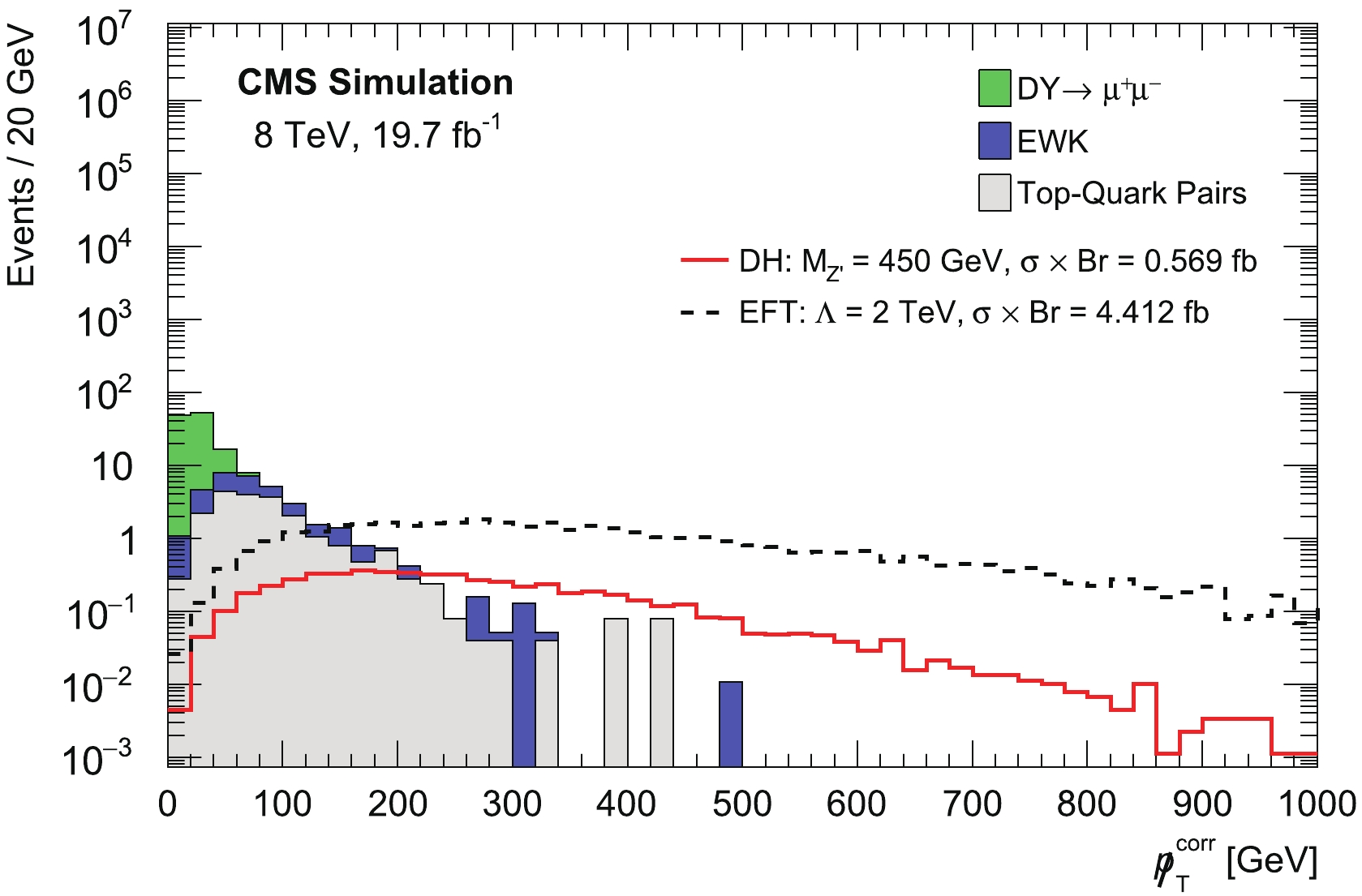# Search for dark matter production in association with the Z' boson at the LHC in pp collisions at ${{\sqrt{s}}}$= 8 TeV using Monte Carlo simulations• This analysis evaluates the possibility of the search for Dark Matter (DM) particles using events with a Z$^{\prime}$heavy gauge boson and a large missing transverse momentum at the Large Hadron Collider (LHC). We consider the muonic decay of Z$^{\prime}$. The analyzed Monte Carlo samples were the Open simulated files produced by the Compact Muon Solenoid (CMS) collaboration for proton-proton collisions, corresponding to an integrated luminosity of the LHC run-I with 19.7 fb $^{-1}$at $\sqrt{s} =$8 TeV. Two scenarios, namely a simplified benchmark scenario, called Dark Higgs, and the effective field theory (EFT) formalism, were used for interpretations. Limits were set on Z$^{\prime}$, dark matter masses, and the cutoff scale of the EFT.
••Get Citation
S. Elgammal, M. A. Loukay, A. Y. Ellithi and M. T. Hussein. Search for dark matter production in association with the Z' boson at the LHC in pp collisions at $\sqrt{s}$= 8 TeV using Monte Carlo simulations[J]. Chinese Physics C. doi: 10.1088/1674-1137/ac061c
S. Elgammal, M. A. Loukay, A. Y. Ellithi and M. T. Hussein. Search for dark matter production in association with the Z' boson at the LHC in pp collisions at $\sqrt{s}$= 8 TeV using Monte Carlo simulations[J]. Chinese Physics C.Milestone
Article Metric

Article Views(287)
Cited by(0)
Policy on re-use
To reuse of Open Access content published by CPC, for content published under the terms of the Creative Commons Attribution 3.0 license (“CC CY”), the users don’t need to request permission to copy, distribute and display the final published version of the article and to create derivative works, subject to appropriate attribution.
###### 通讯作者: 陈斌, bchen63@163.com
• 1.

沈阳化工大学材料科学与工程学院 沈阳 110142

Title:
Email:

## Search for dark matter production in association with the Z' boson at the LHC in pp collisions at ${{\sqrt{s}}}$= 8 TeV using Monte Carlo simulations

###### Corresponding author: S. Elgammal, sherif.elgammal@bue.edu.eg
• 1. Centre for theoretical physics, The British University in Egypt, Cairo, Egypt
• 2. Physics Department, Faculty of Science, Cairo University, Egypt

Abstract: This analysis evaluates the possibility of the search for Dark Matter (DM) particles using events with a Z$^{\prime}$heavy gauge boson and a large missing transverse momentum at the Large Hadron Collider (LHC). We consider the muonic decay of Z$^{\prime}$. The analyzed Monte Carlo samples were the Open simulated files produced by the Compact Muon Solenoid (CMS) collaboration for proton-proton collisions, corresponding to an integrated luminosity of the LHC run-I with 19.7 fb $^{-1}$at $\sqrt{s} =$8 TeV. Two scenarios, namely a simplified benchmark scenario, called Dark Higgs, and the effective field theory (EFT) formalism, were used for interpretations. Limits were set on Z$^{\prime}$, dark matter masses, and the cutoff scale of the EFT.

### HTMLII.   MONO-Z$^{\prime}$ MODELIII.   THE CMS DETECTORIV.   SIMULATED SAMPLESVI.   EVENT SELECTION AND ANALYSIS STRATEGYVII.   SYSTEMATIC UNCERTAINTIESVIII.   RESULTS
Reference (46)
PDF查看关注分享

Top

### 目录/DownLoad:  Full-Size Img  PowerPoint